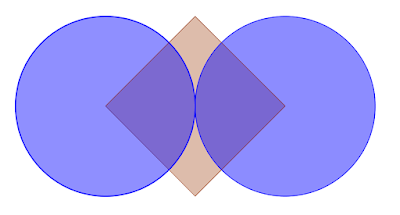## Thursday, July 16, 2015

### 487: The Fifteen Puzzle

Everyone older than ten knows that there is a way to play tic-tac-toe so that you can never lose. Any game that can be played to a draw unless someone makes a newbie's mistake, is boring once you know the secret.

Let's look at this one and see if you can find a strategy for it:

Players alternate writing a number from 1 - 9 (once used, it’s dead). First one with a set of three numbers that sum to 15 wins.

Is there a guaranteed winning strategy for Player 1?
Is there a guaranteed winning strategy for Player 2?

source:
How does his later comment help? "It’s equivalent to tic-tac-toe since any row/col/diagonal in a 3x3 magic square sums to 15, but more mathematically interesting."

### 486: The Bowling Pins Puzzle

Everyone older than ten knows that there is a way to play tic-tac-toe so that you can never lose. Any game that can be played to a draw unless someone makes a newbie's mistake, is boring once you know the secret.

Let's look at this one and see if you can find a strategy for it:

There are thirteen pins and the two players take turns bowling.When bowling, you can knock down any pin or two adjacent pins (but not two that are separated by a space) that you like.  The loser knocks over the last pin.

The gnome has bowled first, knocking down #2. How do you best play this game so that you are guaranteed to win?

If you go first, what is your best play?

source: Loyd's Encyclopedia, 1914.

## Monday, July 13, 2015

### 485: Number Line

On a number line, how many positive integers are closer to 50 than to 100?

source.

### 484: Tempus Fugit

If the time is 10:10, what is the exact angle between the minute hand and hour hand?

### 483: Time and Time Again

How many times per day do the hands make a straight angle? What are some of these times?

How many times per day do the hands make a right angle? What are some of these times?

### 482: Angling for More Time

If the MINUTE hand is on 2, and the hour hand and minute hand make an acute angle, what time could it be?

If the MINUTE hand is on 8, and the hour hand and minute hand make an obtuse angle, what time could it be?

source.

## Saturday, July 11, 2015

### 481: Two Circles and One SquareWhat is the red area?
The two vertices of the square are the centers of two tangent and congruent circles. If the length of a side is 8√2, what is the area of the red part peeping out?

Here is the real question: Does it matter if the circles are congruent, as long as they're tangent and the centers are at the vertices of the square?